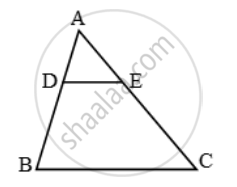# D and E are points on the sides AB and AC respectively of a ∆ABC such that DE || BC and divides ∆ABC into two parts, equal in area. Find - Mathematics

Sum

D and E are points on the sides AB and AC respectively of a ∆ABC such that DE || BC and divides ∆ABC into two parts, equal in area. Find

#### Solution

We have,

Area (∆ADE) = Area (trapezium BCED)

= Area (trapezium BCED) + Area (∆ADE)

⇒ 2 Area (∆ADE) = Area (∆ABC)In ∆ADE and ∆ABC, we have

∠ADE = ∠B [∵ DE || BC ∴ ∠ADE = ∠B (Corresponding angles)] and, ∠A = ∠A [Common]

\Rightarrow \frac{Area\ (\Delta ADE)}{Area\ (\Delta ABC)}=(AD^2)/(AB^2)

(Area(DeltaADE))/(2Area(DeltaADE))=(AD^2)/(AB^2)

\Rightarrow \frac{1}{2}=( \frac{AD}{AB})^{2}\Rightarrow \frac{AD}{AB}=\frac{1}{\sqrt{2}}

⇒ AB = √2 AD AB = √2 (AB – BD)

⇒ (√2 – 1) AB = √2 BD

\Rightarrow \frac{BD}{AB}=\frac{\sqrt{2}-1}{\sqrt{2}}=\frac{2-\sqrt{2}}{2}

Concept: Areas of Similar Triangles
Is there an error in this question or solution?